# Alphanumeric series

## Introduction

Alphanumeric series is a sequence of characters containing either alphabets or numbers or both. In some cases, it may also include special characters like ‘@’,’&’,’*’ etc.

This type of reasoning is a mixed bag of coding-decoding, series-based reasoning and finding positions-based reasoning questions.

Alphanumeric series problems are asked in many competitive exams and in the aptitude round of various companies.

## Arithmetic and Geometric Progression

AP and GP are very important concepts of the alphanumeric series. Some important formulas of Arithmetic and Geometric progression are given below.

### Arithmetic progression(AP)

Arithmetic Progression is a series in which the difference b/w two consecutive terms remain constant.

### Notations in AP

In AP, we will come across three main terms, which are denoted as:

1. Common difference (d)
2. nth Term (an)
3. Sum of the first n terms (Sn)

All three terms represent the property of Arithmetic Progression. We will learn more about these three properties in the next section.

### Common Difference in AP

Suppose, a1, a2, a3, ……………., an is an AP, then; the common difference “ d ” can be obtained as:

d = a2 – a1 = a3 – a2 = ……. = an – an – 1

Where “d” is a common difference. It can be positive, negative or zero.

### First Term of AP

The AP can also be written in terms of common differences, as follows;

a, a + d, a + 2d, a + 3d, a + 4d, ………. ,a + (n – 1) d

where  “a” is the first term of the progression.

### General Form of an A. P

Consider an AP to be: a1, a2, a3, ……………., an.

The general form of this AP will be: a + (n-1)d

### Formula for nth Term of an AP

The formula for finding the n-th term of an AP is:

an = a + (n − 1) × d

Where, a = First term, d = Common difference, n = number of terms and an = nth term

### Sum of N Terms of AP

The sum of the first n terms of an AP can be calculated if the first term and the total number of terms are known. The formula for the sum of AP is given below:

Consider an AP consisting of “n” terms.

S = n/2[2a + (n − 1) × d]

### Sum of AP when the Last Term is Given

Formula to find the sum of AP when first and last terms are given as follows:

S  = n/2 (first term + last term)

### Geometric Progression(GP)

A geometric progression(GP) is a sequence where every term contains a constant ratio to its preceding term.

### General Form of GP

The general form of Geometric Progression is: a, ar, ar2, ar3, ar4,…,an

Where, a = First term, r = common ratio and an = nth term

### General Term or Nth Term of GP

Let a be the first term and r be the common ratio for a G.P.

Then the second term, a2 = a × r = ar

Third term, a3 = a2 × r = ar × r = ar2

Similarly, nth term, an = arn-1

Therefore, the formula to find the nth term of GP is: an = arn-1

Note: nth term is the last term of GP.

### Common Ratio

Consider the sequence a, ar, ar2, ar3,……

First term = a

Second term = ar

Third term = ar2

Similarly nth term, tn = arn-1

Thus, Common ratio = (Any term) / (Preceding term) = tn / tn-1

= (arn – 1 ) /(arn – 2)

= r

### Sum of N term of GP

Suppose a, ar, ar2, ar3, ar4,…,an-1 is the given Geometric Progression.

Then the sum of n terms of GP is given by:

Sn = a + ar + ar2 + ar3 + ar4+…an-1

The formula to find sum of n terms of GP is: Sn = a[(rn-1)/(r-1)] if r ≠ 1

Where a is the first term, r is the common ratio and n is the number of terms

Also, if the common ratio is equal to 1, then the sum of the GP is given by: Sn = na if r=1

## Types of problems in Alphanumeric series

Problems in alphanumeric series are majorly divided into the following three types

### Type 1: Tracking down the next term in the series

To take care of these sorts of issues you need to notice the example of events of numbers and letters in order. Begin searching for designs either from the left or right of the grouping.

Example:

1. Find the next term in the given sequence: 1,3,5,7,_

Ans: 9

Explanation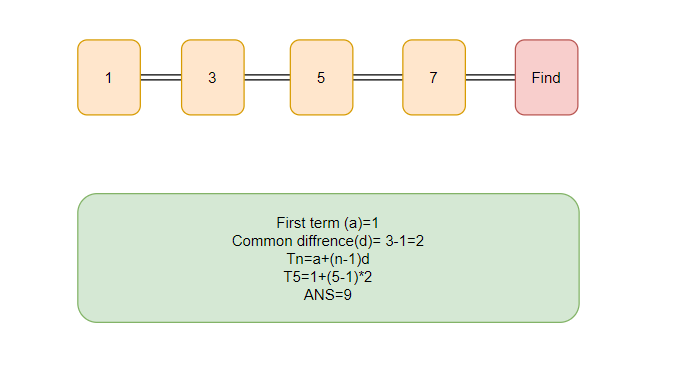Since this sequence is an AP with (first-term = 1 and common-difference = 2)

Applying the formula of nth term of AP, we get

T5=a+(n-1)*d;

T5=1+(5-1)*2=9

### Type 2: Tracking down the missing term

This sort of issue is like the past one. Here you need to notice the example till the missing term and keep following the example up till the finish of the succession.

Example:

Find the missing term in the given sequence: 1,4,7,_,13,16,_

Ans : 10,19

Explanation:

Consider the diagram given below to understand the explanation.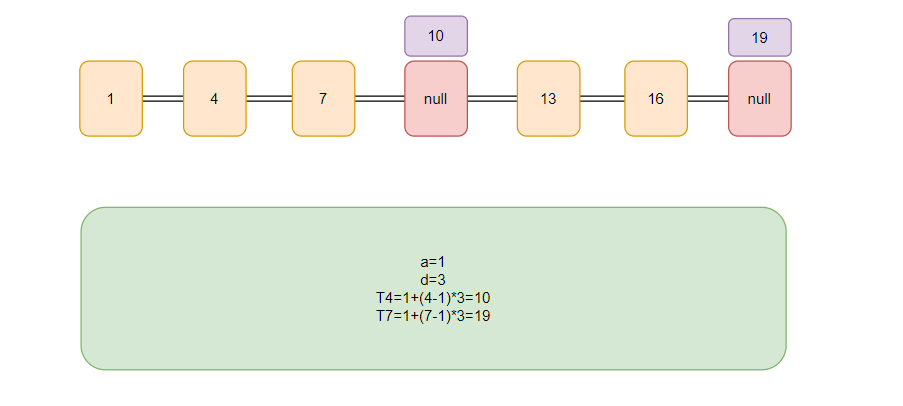From the above image we conclude that the given series is an AP with

First term(a)=1;

Common difference (d)=(7-3)=4;

T4=a+(n-1)d      //substitute n with 4

T4=10

T7=19

### Type 3: Positional inquiries

In this sort of inquiry, we will be given an arrangement and requested to discover specific letters in order or numbers dependent on a specific condition.

Example:

Consider a sequence in which we have to find the missing term 1,A,4,B,2,C,5,D,__  ?

Explanation: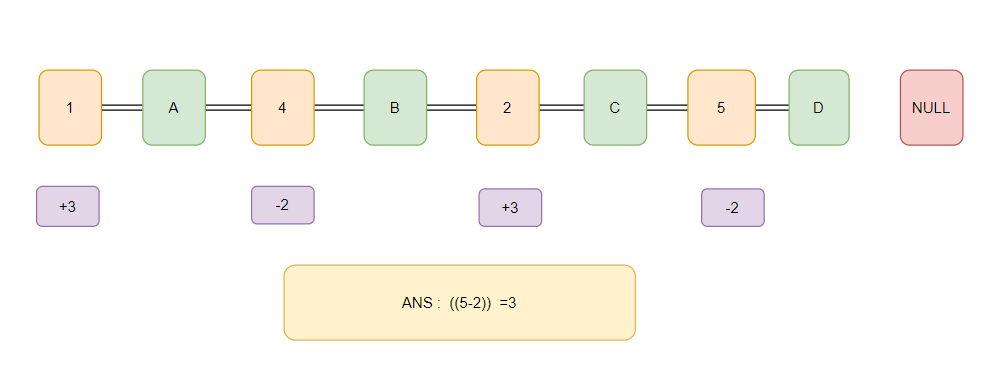## Example’s on Alphanumeric Series

Below are given some examples of alphanumeric series:

Example 1: Find the next term of the given series: 2,A,9,B,6,C,13,D,? (Infosys Hiring 2019)

Solution: Let us understand the explanation of the above example with the help of the diagram below: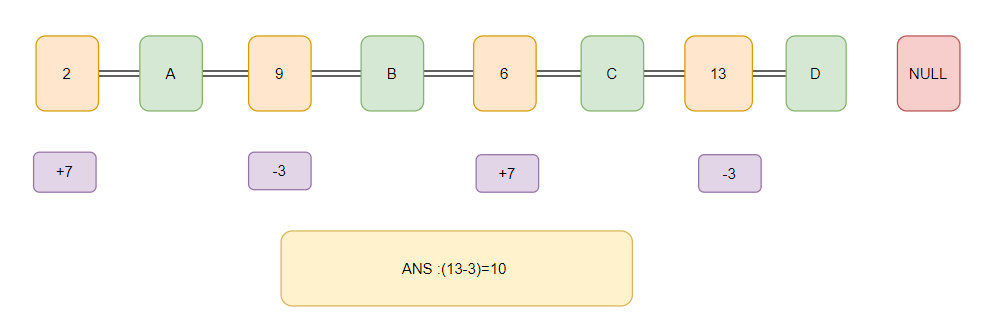From the above image, we conclude that

A number is followed by an alphabet and vice versa. So, We observe that the number pattern goes this way: 2(+7)-->9(-3)-->6(+7)-->13(-3)-->10(the missing value).

Example 2: Consider the sequence ACE? MOQ …… SUW(last term) (Asked in Wipro hiring )?

Solution: Let us understand the explanation of the above example with the help of the diagram below: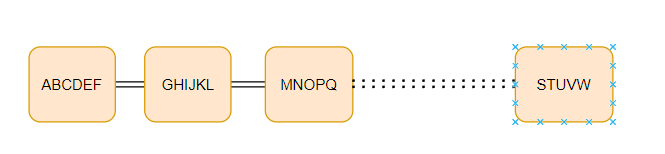From the above image we can conclude that the series move in the given format:

(ABCDE)F(GHIJK)L(MNOPQ)...(STUVW)

### What is an alphanumeric series?

Answer: Alphanumeric series is a sequence of characters containing either alphabets or numbers or both. In some cases, it may also include special characters like ‘@’,’&’,’*’ etc.

### What are the different types of problems on the alphanumeric series?

• Type1- Finding the missing Term
• Type2- Finding the next term
• Type3-Positional Inquiries

### What is an AP?

Answer: AP or arithmetic progression is a series in which the difference b/w two consecutive terms is constant.

### What is GP?

Answer: A geometric progression(GP) is a sequence where every term contains a constant ratio to its preceding term.

### What are the general terms of AP and GP?

Answer: General term of an AP: (Tn)= a+(n-1) *d

General term of a GP: (Tn)= a*r^(n-1)

## Key takeaways

In this blog, we’ve covered the problem of the Alphanumeric Series and discussed how we should solve the problem of the Alphanumeric Series.